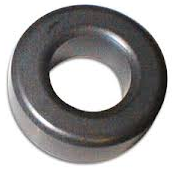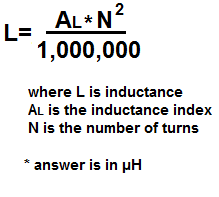﻿ Ferrite Core Inductor Calculator ﻿# Ferrite Core Inductor CalculatorYou must enter values into all 2 form fields to compute result.

Number of Turns:

Inductance Index, AL:

Answer: 0.00µH

The ferrite core inductor calculator calculates the inductance, L, of an inductor, based on the inductance index, AL, and the number of turns, N, in the inductor, according to the above formula.

To use this inductance calculator, a user must enter the value of the inductance index, AL, and the number of turns, N, and click the 'Calculate' button. The inductance output result will then be calculated and automatically displayed. The result of this output value is calculated in unit henry (H).

Related Resources

﻿